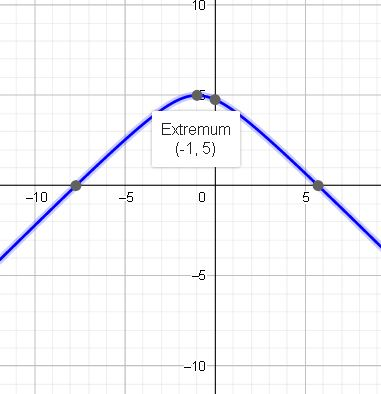# Let function f(x) be defined by f(x) = 7 - sqrt{x^2 + 2x + 5}. (a) Sketch the graph of f(x) by...

## Question:

Let function {eq}f(x) {/eq} be defined by {eq}f(x) = 7 - \sqrt{x^2 + 2x + 5} {/eq}.

(a) Sketch the graph of {eq}f(x) {/eq} by creating a table of points of the graph.

(b) Find the domain and range of {eq}f(x) {/eq}.

(c) Does {eq}f(x) {/eq} preserves any of the symmetries?

A radical function is a type of function that is characterized by having a radical inside the function. In order to fully understand a radical function, we need to simplify first the function and find its important points.

Become a Study.com member to unlock this answer!

We are given the function {eq}f(x)=7\:-\sqrt{\:x^2\:+\:2x\:+\:5} {/eq}.

A. The graph of the function is:B. Based on the graoh, the domain of...What is a Radical Function? - Definition, Equations & Graphs

from

Chapter 7 / Lesson 3
37K